SNAP Library 6.0, Developer Reference  2020-12-09 16:24:20 SNAP, a general purpose, high performance system for analysis and manipulation of large networks
TNGraphMP Class Reference

Directed graph for multi-threaded operations. More...

`#include <graphmp.h>`

Collaboration diagram for TNGraphMP: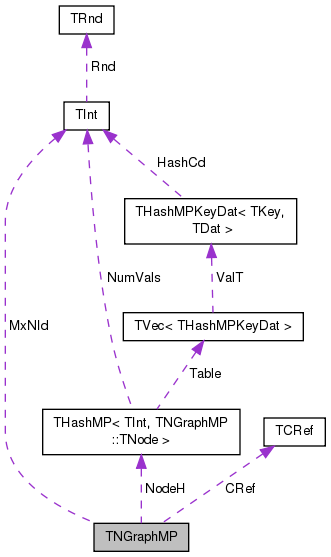[legend]

## Classes

class  TEdgeI
Edge iterator. Only forward iteration (operator++) is supported. More...

class  TNode

class  TNodeI
Node iterator. Only forward iteration (operator++) is supported. More...

## Public Types

typedef TNGraphMP TNet

typedef TPt< TNGraphMPPNet

## Public Member Functions

TNGraphMP ()

TNGraphMP (const int &Nodes, const int &Edges)
Constructor that reserves enough memory for a graph of Nodes nodes and Edges edges. More...

TNGraphMP (const TNGraphMP &Graph)

TNGraphMP (TSIn &SIn)
Constructor that loads the graph from a (binary) stream SIn. More...

void Save (TSOut &SOut) const
Saves the graph to a (binary) stream SOut. More...

bool HasFlag (const TGraphFlag &Flag) const
Allows for run-time checking the type of the graph (see the TGraphFlag for flags). More...

TNGraphMPoperator= (const TNGraphMP &Graph)

int GetNodes () const
Returns the number of nodes in the graph. More...

void SetNodes (const int &Length)
Sets the number of nodes in the graph. More...

Adds a node of ID NId to the graph. More...

Adds a node of ID NId to the graph without performing checks. More...

Adds a node of ID NodeI.GetId() to the graph. More...

int AddNode (const int &NId, const TIntV &InNIdV, const TIntV &OutNIdV)
Adds a node of ID NId to the graph, creates edges to the node from all nodes in vector InNIdV, creates edges from the node to all nodes in vector OutNIdV. More...

int AddNode (const int &NId, const TVecPool< TInt > &Pool, const int &SrcVId, const int &DstVId)
Adds a node of ID NId to the graph, creates edges to the node from all nodes in vector InNIdV in the vector pool Pool, creates edges from the node to all nodes in vector OutNIdVin the vector pool Pool . More...

void DelNode (const int &NId)
Deletes node of ID NId from the graph. More...

void DelNode (const TNode &NodeI)
Deletes node of ID NodeI.GetId() from the graph. More...

bool IsNode (const int &NId) const
Tests whether ID NId is a node. More...

TNodeI BegNI () const
Returns an iterator referring to the first node in the graph. More...

TNodeI EndNI () const
Returns an iterator referring to the past-the-end node in the graph. More...

TNodeI GetNI (const int &NId) const
Returns an iterator referring to the node of ID NId in the graph. More...

int GetMxNId () const
Returns the maximum id of a any node in the graph. More...

int Reserved () const

int GetEdges () const
Returns the number of edges in the graph. More...

int AddEdge (const int &SrcNId, const int &DstNId)
Adds an edge from node IDs SrcNId to node DstNId to the graph. More...

int AddEdgeUnchecked (const int &SrcNId, const int &DstNId)
Adds an edge from node IDs SrcNId to node DstNId to the graph without performing checks. More...

Adds an edge from EdgeI.GetSrcNId() to EdgeI.GetDstNId() to the graph. More...

int AddOutEdge1 (int &SrcIdx, const int &SrcNId, const int &DstNId)

int AddInEdge1 (int &DstIdx, const int &SrcNId, const int &DstNId)

void AddOutEdge2 (const int &SrcNId, const int &DstNId)

void AddInEdge2 (const int &SrcNId, const int &DstNId)

void AddNodeWithEdges (const TInt &NId, TIntV &InNIdV, TIntV &OutNIdV)
Adds a node of ID NId to the graph, creates edges to the node from all nodes in vector InNIdV, creates edges from the node to all nodes in vector OutNIdV. More...

void DelEdge (const int &SrcNId, const int &DstNId, const bool &IsDir=true)
Deletes an edge from node IDs SrcNId to DstNId from the graph. More...

bool IsEdge (const int &SrcNId, const int &DstNId, const bool &IsDir=true) const
Tests whether an edge from node IDs SrcNId to DstNId exists in the graph. More...

TEdgeI BegEI () const
Returns an iterator referring to the first edge in the graph. More...

TEdgeI EndEI () const
Returns an iterator referring to the past-the-end edge in the graph. More...

TEdgeI GetEI (const int &SrcNId, const int &DstNId) const
Returns an iterator referring to edge (SrcNId, DstNId) in the graph. More...

int GetRndNId (TRnd &Rnd=TInt::Rnd)
Returns an ID of a random node in the graph. More...

TNodeI GetRndNI (TRnd &Rnd=TInt::Rnd)
Returns an interator referring to a random node in the graph. More...

void GetNIdV (TIntV &NIdV) const
Gets a vector IDs of all nodes in the graph. More...

bool Empty () const
Tests whether the graph is empty (has zero nodes). More...

void Clr ()
Deletes all nodes and edges from the graph. More...

void Reserve (const int &Nodes, const int &Edges)
Reserves memory for a graph of Nodes nodes and Edges edges. More...

void ReserveNodeDegs (const int &Idx, const int &InDeg, const int &OutDeg)
Reserves memory for node Idx having InDeg in-edges and OutDeg out-edges. More...

void ReserveNIdInDeg (const int &NId, const int &InDeg)
Reserves memory for node ID NId having InDeg in-edges. More...

void ReserveNIdOutDeg (const int &NId, const int &OutDeg)
Reserves memory for node ID NId having OutDeg out-edges. More...

void SortEdges (const int &Idx, const int &InDeg, const int &OutDeg)
Sorts in-edges and out-edges. More...

Sorts the adjacency lists of each node. More...

Defragments the graph. More...

bool IsOk (const bool &ThrowExcept=true) const
Checks the graph data structure for internal consistency. More...

void Dump (FILE *OutF=stdout) const
Print the graph in a human readable form to an output stream OutF. More...

## Static Public Member Functions

static PNGraphMP New ()
Static constructor that returns a pointer to the graph. Call: PNGraphMP Graph = TNGraphMP::New(). More...

static PNGraphMP New (const int &Nodes, const int &Edges)
Static constructor that returns a pointer to the graph and reserves enough memory for Nodes nodes and Edges edges. More...

Static constructor that loads the graph from a stream SIn and returns a pointer to it. More...

static PNGraphMP GetSmallGraph ()
Returns a small graph on 5 nodes and 6 edges. More...

## Private Member Functions

TNodeGetNode (const int &NId)

const TNodeGetNode (const int &NId) const

## Private Attributes

TCRef CRef

TInt MxNId

THashMP< TInt, TNodeNodeH

## Friends

class TPt< TNGraphMP >

class TNGraphMPMtx

## Detailed Description

Node IDs can be arbitrary non-negative integers. Nodes and edges have no attributes/data associated with them. There is at most one directed edge from one source node to a destination node. There can be an edge between the same pair of nodes in the opposite direction. Self loops (one per node) are allowed but multiple (parallel) edges are not. The directed graph data structure is implemented using sorted adjacency lists. This means adding a node takes constant time, while adding an edge takes linear time (since adjacency list is kept sorted) in the node degree. Accessing arbitrary node takes constant time and accessing any edge takes logarithmic time in the node degree.

Definition at line 27 of file graphmp.h.

## Member Typedef Documentation

 typedef TPt TNGraphMP::PNet

Definition at line 30 of file graphmp.h.

 typedef TNGraphMP TNGraphMP::TNet

Definition at line 29 of file graphmp.h.

## Constructor & Destructor Documentation

 TNGraphMP::TNGraphMP ( )
inline

Definition at line 132 of file graphmp.h.

132 : CRef(), MxNId(0), NodeH() { }
TCRef CRef
Definition: graphmp.h:125
THashMP< TInt, TNode > NodeH
Definition: graphmp.h:127
TInt MxNId
Definition: graphmp.h:126

Here is the caller graph for this function:TNGraphMP::TNGraphMP ( const int & Nodes, const int & Edges )
inlineexplicit

Constructor that reserves enough memory for a graph of Nodes nodes and Edges edges.

Definition at line 134 of file graphmp.h.

References Reserve().

134 : MxNId(0) { Reserve(Nodes, Edges); }
void Reserve(const int &Nodes, const int &Edges)
Reserves memory for a graph of Nodes nodes and Edges edges.
Definition: graphmp.h:259
TInt MxNId
Definition: graphmp.h:126

Here is the call graph for this function: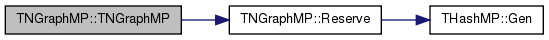TNGraphMP::TNGraphMP ( const TNGraphMP & Graph )
inline

Definition at line 135 of file graphmp.h.

135 : MxNId(Graph.MxNId), NodeH(Graph.NodeH) { }
THashMP< TInt, TNode > NodeH
Definition: graphmp.h:127
TInt MxNId
Definition: graphmp.h:126
 TNGraphMP::TNGraphMP ( TSIn & SIn )
inline

Constructor that loads the graph from a (binary) stream SIn.

Definition at line 137 of file graphmp.h.

137 : MxNId(SIn), NodeH(SIn) { }
THashMP< TInt, TNode > NodeH
Definition: graphmp.h:127
TInt MxNId
Definition: graphmp.h:126

## Member Function Documentation

 int TNGraphMP::AddEdge ( const int & SrcNId, const int & DstNId )

Adds an edge from node IDs SrcNId to node DstNId to the graph.

If the edge already exists return -2. If the edge was successfully added return -1. Normally the function should return an ID of the edge added but since edges in TNGraphMP have no IDs we return -1. Function aborts if SrcNId or DstNId are not nodes in the graph.

Definition at line 97 of file graphmp.cpp.

97  {
98  IAssertR(IsNode(SrcNId) && IsNode(DstNId), TStr::Fmt("%d or %d not a node.", SrcNId, DstNId).CStr());
99  //IAssert(! IsEdge(SrcNId, DstNId));
100  if (IsEdge(SrcNId, DstNId)) { return -2; }
103  return -1; // edge id
104 }
#define IAssertR(Cond, Reason)
Definition: bd.h:265
TIntV OutNIdV
Definition: graphmp.h:35
TSizeTy AddSorted(const TVal &Val, const bool &Asc=true, const TSizeTy &_MxVals=-1)
Adds element Val to a sorted vector.
Definition: ds.h:1117
TIntV InNIdV
Definition: graphmp.h:35
bool IsNode(const int &NId) const
Tests whether ID NId is a node.
Definition: graphmp.h:195
static TStr Fmt(const char *FmtStr,...)
Definition: dt.cpp:1599
bool IsEdge(const int &SrcNId, const int &DstNId, const bool &IsDir=true) const
Tests whether an edge from node IDs SrcNId to DstNId exists in the graph.
Definition: graphmp.cpp:130
TNode & GetNode(const int &NId)
Definition: graphmp.h:129

Here is the call graph for this function: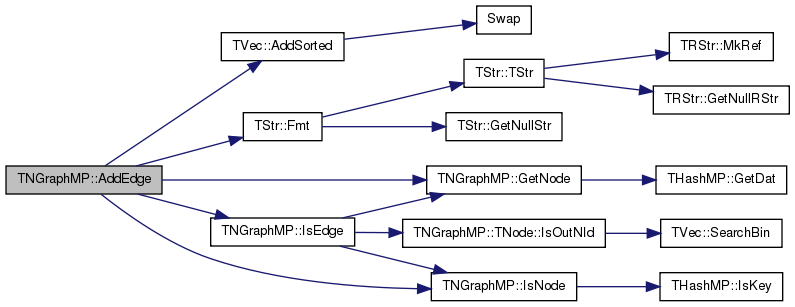int TNGraphMP::AddEdge ( const TEdgeI & EdgeI )
inline

Adds an edge from EdgeI.GetSrcNId() to EdgeI.GetDstNId() to the graph.

Definition at line 221 of file graphmp.h.

221 { return AddEdge(EdgeI.GetSrcNId(), EdgeI.GetDstNId()); }
int AddEdge(const int &SrcNId, const int &DstNId)
Adds an edge from node IDs SrcNId to node DstNId to the graph.
Definition: graphmp.cpp:97

Here is the call graph for this function:Here is the caller graph for this function:int TNGraphMP::AddEdgeUnchecked ( const int & SrcNId, const int & DstNId )

Adds an edge from node IDs SrcNId to node DstNId to the graph without performing checks.

Definition at line 106 of file graphmp.cpp.

106  {
109  return -1; // edge id
110 }
TIntV OutNIdV
Definition: graphmp.h:35
TIntV InNIdV
Definition: graphmp.h:35
Adds a new element at the end of the vector, after its current last element.
Definition: ds.h:602
TNode & GetNode(const int &NId)
Definition: graphmp.h:129

Here is the call graph for this function:int TNGraphMP::AddInEdge1 ( int & DstIdx, const int & SrcNId, const int & DstNId )

Definition at line 253 of file graphmp.cpp.

References THashMP< TKey, TDat, THashFunc >::AddKey12(), and NodeH.

253  {
254  bool Found;
255  int DstKeyId;
256
257  //DstKeyId = NodeH.AddKey2(DstIdx, DstNId, Found);
258  DstKeyId = NodeH.AddKey12(DstIdx, DstNId, Found);
259  if (!Found) {
260  NodeH[DstKeyId] = TNode(DstNId);
261  //MxNId = TMath::Mx(DstNId+1, MxNId());
262  }
265
266  // TODO:RS, edge lists need to be sorted at the end
267
268  DstIdx = DstKeyId;
269  return Found;
270 }
THashMP< TInt, TNode > NodeH
Definition: graphmp.h:127
int AddKey12(const int &Idx, const TKey &Key, bool &Found)
Definition: hashmp.h:307

Here is the call graph for this function: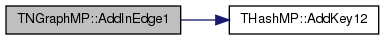void TNGraphMP::AddInEdge2 ( const int & SrcNId, const int & DstNId )

Definition at line 276 of file graphmp.cpp.

References THashMP< TKey, TDat, THashFunc >::GetKeyId(), and NodeH.

276  {
278 }
THashMP< TInt, TNode > NodeH
Definition: graphmp.h:127
int GetKeyId(const TKey &Key) const
Definition: hashmp.h:459

Here is the call graph for this function: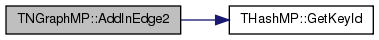int TNGraphMP::AddNode ( int NId = `-1` )

Adds a node of ID NId to the graph.

Returns the ID of the node being added. If NId is -1, node ID is automatically assigned. Aborts, if a node with ID NId already exists.

Definition at line 10 of file graphmp.cpp.

10  {
11  if (NId == -1) {
12  NId = MxNId; MxNId++;
13  } else {
14  IAssertR(!IsNode(NId), TStr::Fmt("NodeId %d already exists", NId));
15  MxNId = TMath::Mx(NId+1, MxNId());
16  }
18  return NId;
19 }
#define IAssertR(Cond, Reason)
Definition: bd.h:265
static const T & Mx(const T &LVal, const T &RVal)
Definition: xmath.h:32
bool IsNode(const int &NId) const
Tests whether ID NId is a node.
Definition: graphmp.h:195
THashMP< TInt, TNode > NodeH
Definition: graphmp.h:127
static TStr Fmt(const char *FmtStr,...)
Definition: dt.cpp:1599
TInt MxNId
Definition: graphmp.h:126
Definition: hashmp.h:181

Here is the call graph for this function: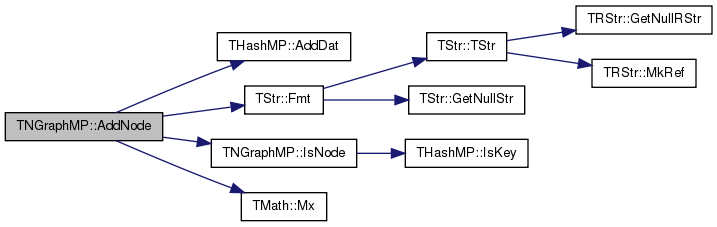int TNGraphMP::AddNode ( const TNodeI & NodeId )
inline

Adds a node of ID NodeI.GetId() to the graph.

Definition at line 166 of file graphmp.h.

Adds a node of ID NId to the graph.
Definition: graphmp.cpp:10

Here is the call graph for this function:Here is the caller graph for this function:int TNGraphMP::AddNode ( const int & NId, const TIntV & InNIdV, const TIntV & OutNIdV )

Adds a node of ID NId to the graph, creates edges to the node from all nodes in vector InNIdV, creates edges from the node to all nodes in vector OutNIdV.

Returns the ID of the node being added. If NId is -1, node ID is automatically assigned. Aborts, if a node with ID NId already exists.

The operation can create inconsistent graphs when the neighboring nodes in vectors InNIdV and OutNIdV do not exist. Use TNGraphMP::IsOk to check that the resulting graph is consistent after the operation.

Definition at line 30 of file graphmp.cpp.

30  {
31  int NewNId;
32  if (NId == -1) {
33  NewNId = MxNId; MxNId++;
34  } else {
35  IAssertR(!IsNode(NId), TStr::Fmt("NodeId %d already exists", NId));
36  NewNId = NId;
37  MxNId = TMath::Mx(NewNId+1, MxNId());
38  }
40  Node.Id = NewNId;
41  Node.InNIdV = InNIdV;
42  Node.OutNIdV = OutNIdV;
43  Node.InNIdV.Sort();
44  Node.OutNIdV.Sort();
45  return NewNId;
46 }
#define IAssertR(Cond, Reason)
Definition: bd.h:265
static const T & Mx(const T &LVal, const T &RVal)
Definition: xmath.h:32
void Sort(const bool &Asc=true)
Sorts the elements of the vector.
Definition: ds.h:1318
bool IsNode(const int &NId) const
Tests whether ID NId is a node.
Definition: graphmp.h:195
THashMP< TInt, TNode > NodeH
Definition: graphmp.h:127
static TStr Fmt(const char *FmtStr,...)
Definition: dt.cpp:1599
TInt MxNId
Definition: graphmp.h:126
Definition: hashmp.h:181

Here is the call graph for this function: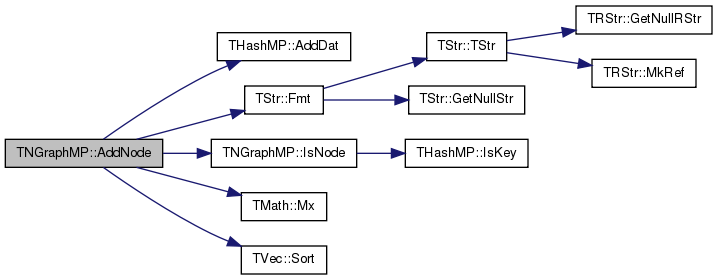int TNGraphMP::AddNode ( const int & NId, const TVecPool< TInt > & Pool, const int & SrcVId, const int & DstVId )

Adds a node of ID NId to the graph, creates edges to the node from all nodes in vector InNIdV in the vector pool Pool, creates edges from the node to all nodes in vector OutNIdVin the vector pool Pool .

Returns the ID of the node being added. If NId is -1, node ID is automatically assigned. Aborts, if a node with ID NId already exists.

The operation can create inconsistent graphs when the neighboring nodes stored in the Pool vector are not explicitly added to the graph. Use TNGraphMP::IsOk to check that the resulting graph is consistent.

Definition at line 50 of file graphmp.cpp.

50  {
51  int NewNId;
52  if (NId == -1) {
53  NewNId = MxNId; MxNId++;
54  } else {
55  IAssertR(!IsNode(NId), TStr::Fmt("NodeId %d already exists", NId));
56  NewNId = NId;
57  MxNId = TMath::Mx(NewNId+1, MxNId());
58  }
60  Node.Id = NewNId;
61  Node.InNIdV.GenExt(Pool.GetValVPt(SrcVId), Pool.GetVLen(SrcVId));
62  Node.OutNIdV.GenExt(Pool.GetValVPt(DstVId), Pool.GetVLen(DstVId));
63  Node.InNIdV.Sort();
64  Node.OutNIdV.Sort();
65  return NewNId;
66 }
#define IAssertR(Cond, Reason)
Definition: bd.h:265
static const T & Mx(const T &LVal, const T &RVal)
Definition: xmath.h:32
TVal * GetValVPt(const int &VId) const
Returns pointer to the first element of the vector with id VId.
Definition: ds.h:1731
int GetVLen(const int &VId) const
Returns the number of elements in the vector with id VId.
Definition: ds.h:1729
bool IsNode(const int &NId) const
Tests whether ID NId is a node.
Definition: graphmp.h:195
THashMP< TInt, TNode > NodeH
Definition: graphmp.h:127
static TStr Fmt(const char *FmtStr,...)
Definition: dt.cpp:1599
TInt MxNId
Definition: graphmp.h:126
Definition: hashmp.h:181

Here is the call graph for this function: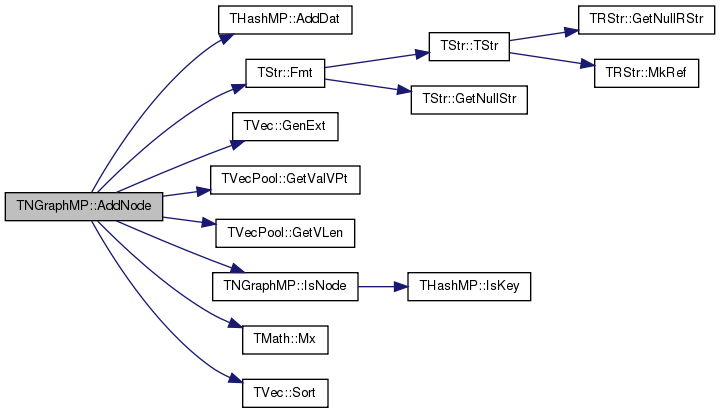int TNGraphMP::AddNodeUnchecked ( int NId = `-1` )

Adds a node of ID NId to the graph without performing checks.

Definition at line 21 of file graphmp.cpp.

References THashMP< TKey, TDat, THashFunc >::AddDat(), IsNode(), TMath::Mx(), MxNId, and NodeH.

21  {
22  if (IsNode(NId)) { return NId;}
23  MxNId = TMath::Mx(NId+1, MxNId());
25  return NId;
26 }
static const T & Mx(const T &LVal, const T &RVal)
Definition: xmath.h:32
bool IsNode(const int &NId) const
Tests whether ID NId is a node.
Definition: graphmp.h:195
THashMP< TInt, TNode > NodeH
Definition: graphmp.h:127
TInt MxNId
Definition: graphmp.h:126
Definition: hashmp.h:181

Here is the call graph for this function:void TNGraphMP::AddNodeWithEdges ( const TInt & NId, TIntV & InNIdV, TIntV & OutNIdV )

Adds a node of ID NId to the graph, creates edges to the node from all nodes in vector InNIdV, creates edges from the node to all nodes in vector OutNIdV.

Definition at line 282 of file graphmp.cpp.

282  {
283  int NodeIdx = abs((NId.GetPrimHashCd()) % Reserved());
284  int NodeKeyId = NodeH.AddKey13(NodeIdx, NId);
285  NodeH[NodeKeyId] = TNode(NId);
286  NodeH[NodeKeyId].InNIdV.MoveFrom(InNIdV);
287  NodeH[NodeKeyId].OutNIdV.MoveFrom(OutNIdV);
288 }
int GetPrimHashCd() const
Definition: dt.h:1171
THashMP< TInt, TNode > NodeH
Definition: graphmp.h:127
int AddKey13(const int &Idx, const TKey &Key)
Definition: hashmp.h:358
int Reserved() const
Definition: graphmp.h:206

Here is the call graph for this function:int TNGraphMP::AddOutEdge1 ( int & SrcIdx, const int & SrcNId, const int & DstNId )

Definition at line 234 of file graphmp.cpp.

References THashMP< TKey, TDat, THashFunc >::AddKey12(), and NodeH.

234  {
235  bool Found;
236  int SrcKeyId;
237
238  //SrcKeyId = NodeH.AddKey2(SrcIdx, SrcNId, Found);
239  SrcKeyId = NodeH.AddKey12(SrcIdx, SrcNId, Found);
240  if (!Found) {
241  NodeH[SrcKeyId] = TNode(SrcNId);
242  //MxNId = TMath::Mx(SrcNId+1, MxNId());
243  }
246
247  // TODO:RS, edge lists need to be sorted at the end
248
249  SrcIdx = SrcKeyId;
250  return Found;
251 }
THashMP< TInt, TNode > NodeH
Definition: graphmp.h:127
int AddKey12(const int &Idx, const TKey &Key, bool &Found)
Definition: hashmp.h:307

Here is the call graph for this function: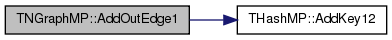void TNGraphMP::AddOutEdge2 ( const int & SrcNId, const int & DstNId )

Definition at line 272 of file graphmp.cpp.

References THashMP< TKey, TDat, THashFunc >::GetKeyId(), and NodeH.

272  {
274 }
THashMP< TInt, TNode > NodeH
Definition: graphmp.h:127
int GetKeyId(const TKey &Key) const
Definition: hashmp.h:459

Here is the call graph for this function: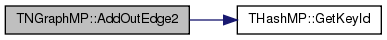TEdgeI TNGraphMP::BegEI ( ) const
inline

Returns an iterator referring to the first edge in the graph.

Definition at line 239 of file graphmp.h.

References BegNI(), EndNI(), and TNGraphMP::TNodeI::GetOutDeg().

239 { TNodeI NI=BegNI(); while(NI<EndNI() && NI.GetOutDeg()==0){NI++;} return TEdgeI(NI, EndNI()); }
TNodeI BegNI() const
Returns an iterator referring to the first node in the graph.
Definition: graphmp.h:197
TNodeI EndNI() const
Returns an iterator referring to the past-the-end node in the graph.
Definition: graphmp.h:199
int GetOutDeg() const
Returns out-degree of the current node.
Definition: graphmp.h:78

Here is the call graph for this function: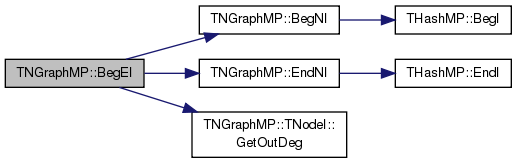TNodeI TNGraphMP::BegNI ( ) const
inline

Returns an iterator referring to the first node in the graph.

Definition at line 197 of file graphmp.h.

References THashMP< TKey, TDat, THashFunc >::BegI().

197 { return TNodeI(NodeH.BegI()); }
THashMP< TInt, TNode > NodeH
Definition: graphmp.h:127
TIter BegI() const
Definition: hashmp.h:153

Here is the call graph for this function: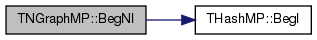Here is the caller graph for this function:void TNGraphMP::Clr ( )
inline

Deletes all nodes and edges from the graph.

Definition at line 257 of file graphmp.h.

References THashMP< TKey, TDat, THashFunc >::Clr().

257 { MxNId=0; NodeH.Clr(); }
THashMP< TInt, TNode > NodeH
Definition: graphmp.h:127
TInt MxNId
Definition: graphmp.h:126
void Clr(const bool &DoDel=true)
Definition: hashmp.h:474

Here is the call graph for this function: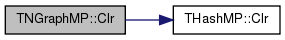void TNGraphMP::Defrag ( const bool & OnlyNodeLinks = `false` )

Defragments the graph.

After performing many node and edge insertions and deletions to a graph, the graph data structure will be fragmented in memory. This function compacts down the graph data structure and frees unneeded memory.

Definition at line 149 of file graphmp.cpp.

149  {
150 #if 0
151  for (int n = NodeH.FFirstKeyId(); NodeH.FNextKeyId(n); ) {
152  TNode& Node = NodeH[n];
153  Node.InNIdV.Pack(); Node.OutNIdV.Pack();
154  }
155  if (! OnlyNodeLinks && ! NodeH.IsKeyIdEqKeyN()) { NodeH.Defrag(); }
156 #endif
157 }
void Pack()
Definition: hashmp.h:218
bool FNextKeyId(int &KeyId) const
Definition: hashmp.h:484
THashMP< TInt, TNode > NodeH
Definition: graphmp.h:127
int FFirstKeyId() const
Definition: hashmp.h:207

Here is the call graph for this function:void TNGraphMP::DelEdge ( const int & SrcNId, const int & DstNId, const bool & IsDir = `true` )

Deletes an edge from node IDs SrcNId to DstNId from the graph.

If the edge (SrcNId, DstNId) does not exist in the graph function still completes. But the function aborts if SrcNId or DstNId are not nodes in the graph.

Definition at line 112 of file graphmp.cpp.

112  {
113  IAssertR(IsNode(SrcNId) && IsNode(DstNId), TStr::Fmt("%d or %d not a node.", SrcNId, DstNId).CStr());
114  { TNode& N = GetNode(SrcNId);
115  const int n = N.OutNIdV.SearchBin(DstNId);
116  if (n!= -1) { N.OutNIdV.Del(n); } }
117  { TNode& N = GetNode(DstNId);
118  const int n = N.InNIdV.SearchBin(SrcNId);
119  if (n!= -1) { N.InNIdV.Del(n); } }
120  if (! IsDir) {
121  { TNode& N = GetNode(SrcNId);
122  const int n = N.InNIdV.SearchBin(DstNId);
123  if (n!= -1) { N.InNIdV.Del(n); } }
124  { TNode& N = GetNode(DstNId);
125  const int n = N.OutNIdV.SearchBin(SrcNId);
126  if (n!= -1) { N.OutNIdV.Del(n); } }
127  }
128 }
#define IAssertR(Cond, Reason)
Definition: bd.h:265
bool IsNode(const int &NId) const
Tests whether ID NId is a node.
Definition: graphmp.h:195
static TStr Fmt(const char *FmtStr,...)
Definition: dt.cpp:1599
TNode & GetNode(const int &NId)
Definition: graphmp.h:129

Here is the call graph for this function: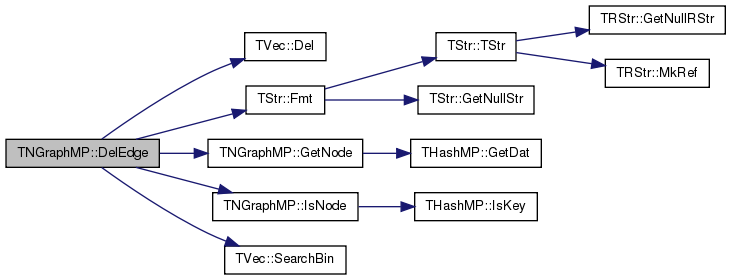void TNGraphMP::DelNode ( const int & NId )

Deletes node of ID NId from the graph.

If the node of ID NId does not exist the function aborts.

Definition at line 68 of file graphmp.cpp.

68  {
69 #if 0
70  { TNode& Node = GetNode(NId);
71  for (int e = 0; e < Node.GetOutDeg(); e++) {
72  const int nbr = Node.GetOutNId(e);
73  if (nbr == NId) { continue; }
74  TNode& N = GetNode(nbr);
75  const int n = N.InNIdV.SearchBin(NId);
76  if (n!= -1) { N.InNIdV.Del(n); }
77  }
78  for (int e = 0; e < Node.GetInDeg(); e++) {
79  const int nbr = Node.GetInNId(e);
80  if (nbr == NId) { continue; }
81  TNode& N = GetNode(nbr);
82  const int n = N.OutNIdV.SearchBin(NId);
83  if (n!= -1) { N.OutNIdV.Del(n); }
84  } }
85  NodeH.DelKey(NId);
86 #endif
87 }
THashMP< TInt, TNode > NodeH
Definition: graphmp.h:127
TNode & GetNode(const int &NId)
Definition: graphmp.h:129

Here is the call graph for this function: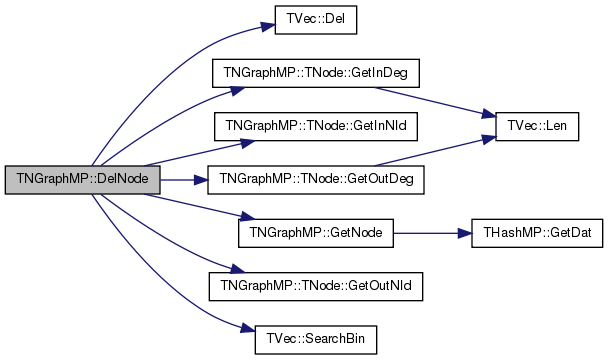void TNGraphMP::DelNode ( const TNode & NodeI )
inline

Deletes node of ID NodeI.GetId() from the graph.

Definition at line 193 of file graphmp.h.

References DelNode(), and TNGraphMP::TNode::GetId().

Referenced by DelNode().

193 { DelNode(NodeI.GetId()); }
void DelNode(const int &NId)
Deletes node of ID NId from the graph.
Definition: graphmp.cpp:68

Here is the call graph for this function:Here is the caller graph for this function: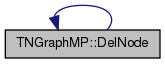void TNGraphMP::Dump ( FILE * OutF = `stdout` ) const

Print the graph in a human readable form to an output stream OutF.

Definition at line 206 of file graphmp.cpp.

206  {
207  const int NodePlaces = (int) ceil(log10((double) GetNodes()));
208  fprintf(OutF, "-------------------------------------------------\nDirected Node Graph: nodes: %d, edges: %d\n", GetNodes(), GetEdges());
209  for (int N = NodeH.FFirstKeyId(); NodeH.FNextKeyId(N); ) {
210  const TNode& Node = NodeH[N];
211  fprintf(OutF, " %*d]\n", NodePlaces, Node.GetId());
212  fprintf(OutF, " in [%d]", Node.GetInDeg());
213  for (int edge = 0; edge < Node.GetInDeg(); edge++) {
214  fprintf(OutF, " %*d", NodePlaces, Node.GetInNId(edge)); }
215  fprintf(OutF, "\n out[%d]", Node.GetOutDeg());
216  for (int edge = 0; edge < Node.GetOutDeg(); edge++) {
217  fprintf(OutF, " %*d", NodePlaces, Node.GetOutNId(edge)); }
218  fprintf(OutF, "\n");
219  }
220  fprintf(OutF, "\n");
221 }
int GetEdges() const
Returns the number of edges in the graph.
Definition: graphmp.cpp:89
bool FNextKeyId(int &KeyId) const
Definition: hashmp.h:484
THashMP< TInt, TNode > NodeH
Definition: graphmp.h:127
int FFirstKeyId() const
Definition: hashmp.h:207
int GetNodes() const
Returns the number of nodes in the graph.
Definition: graphmp.h:154

Here is the call graph for this function: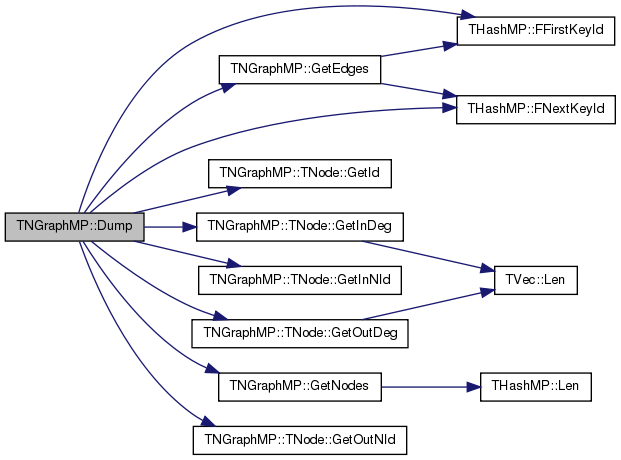bool TNGraphMP::Empty ( ) const
inline

Tests whether the graph is empty (has zero nodes).

Definition at line 255 of file graphmp.h.

References GetNodes().

255 { return GetNodes()==0; }
int GetNodes() const
Returns the number of nodes in the graph.
Definition: graphmp.h:154

Here is the call graph for this function: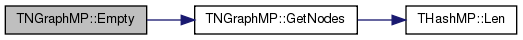TEdgeI TNGraphMP::EndEI ( ) const
inline

Returns an iterator referring to the past-the-end edge in the graph.

Definition at line 241 of file graphmp.h.

References EndNI().

241 { return TEdgeI(EndNI(), EndNI()); }
TNodeI EndNI() const
Returns an iterator referring to the past-the-end node in the graph.
Definition: graphmp.h:199

Here is the call graph for this function: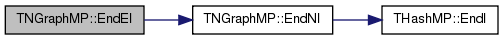TNodeI TNGraphMP::EndNI ( ) const
inline

Returns an iterator referring to the past-the-end node in the graph.

Definition at line 199 of file graphmp.h.

References THashMP< TKey, TDat, THashFunc >::EndI().

Referenced by BegEI(), EndEI(), GetEI(), and SortNodeAdjV().

199 { return TNodeI(NodeH.EndI()); }
TIter EndI() const
Definition: hashmp.h:156
THashMP< TInt, TNode > NodeH
Definition: graphmp.h:127

Here is the call graph for this function: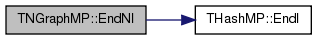Here is the caller graph for this function: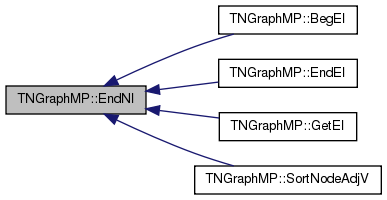int TNGraphMP::GetEdges ( ) const

Returns the number of edges in the graph.

Definition at line 89 of file graphmp.cpp.

Referenced by Dump().

89  {
90  int edges=0;
91  for (int N=NodeH.FFirstKeyId(); NodeH.FNextKeyId(N); ) {
92  edges+=NodeH[N].GetOutDeg();
93  }
94  return edges;
95 }
bool FNextKeyId(int &KeyId) const
Definition: hashmp.h:484
THashMP< TInt, TNode > NodeH
Definition: graphmp.h:127
int FFirstKeyId() const
Definition: hashmp.h:207

Here is the call graph for this function:Here is the caller graph for this function: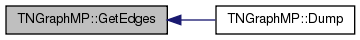TNGraphMP::TEdgeI TNGraphMP::GetEI ( const int & SrcNId, const int & DstNId ) const

Returns an iterator referring to edge (SrcNId, DstNId) in the graph.

Definition at line 136 of file graphmp.cpp.

References EndNI(), GetNI(), IAssert, and TNGraphMP::TNodeI::NodeHI.

136  {
137  const TNodeI SrcNI = GetNI(SrcNId);
138  const int NodeN = SrcNI.NodeHI.GetDat().OutNIdV.SearchBin(DstNId);
139  IAssert(NodeN != -1);
140  return TEdgeI(SrcNI, EndNI(), NodeN);
141 }
#define IAssert(Cond)
Definition: bd.h:262
TNodeI GetNI(const int &NId) const
Returns an iterator referring to the node of ID NId in the graph.
Definition: graphmp.h:201
TNodeI EndNI() const
Returns an iterator referring to the past-the-end node in the graph.
Definition: graphmp.h:199

Here is the call graph for this function:int TNGraphMP::GetMxNId ( ) const
inline

Returns the maximum id of a any node in the graph.

Definition at line 205 of file graphmp.h.

References MxNId.

205 { return MxNId; }
TInt MxNId
Definition: graphmp.h:126
 TNodeI TNGraphMP::GetNI ( const int & NId ) const
inline

Returns an iterator referring to the node of ID NId in the graph.

Definition at line 201 of file graphmp.h.

References THashMP< TKey, TDat, THashFunc >::GetI().

Referenced by GetEI(), and GetRndNI().

201 { return TNodeI(NodeH.GetI(NId)); }
TIter GetI(const TKey &Key) const
Definition: hashmp.h:158
THashMP< TInt, TNode > NodeH
Definition: graphmp.h:127

Here is the call graph for this function: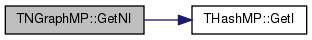Here is the caller graph for this function:void TNGraphMP::GetNIdV ( TIntV & NIdV ) const

Gets a vector IDs of all nodes in the graph.

Definition at line 143 of file graphmp.cpp.

143  {
144  NIdV.Gen(GetNodes(), 0);
145  for (int N=NodeH.FFirstKeyId(); NodeH.FNextKeyId(N); ) {
147 }
bool FNextKeyId(int &KeyId) const
Definition: hashmp.h:484
THashMP< TInt, TNode > NodeH
Definition: graphmp.h:127
int FFirstKeyId() const
Definition: hashmp.h:207
int GetNodes() const
Returns the number of nodes in the graph.
Definition: graphmp.h:154
void Gen(const TSizeTy &_Vals)
Constructs a vector (an array) of _Vals elements.
Definition: ds.h:523
Adds a new element at the end of the vector, after its current last element.
Definition: ds.h:602
const TKey & GetKey(const int &KeyId) const
Definition: hashmp.h:185

Here is the call graph for this function: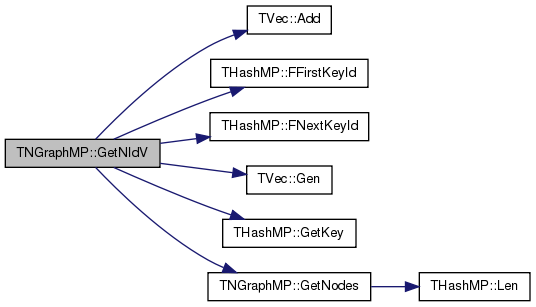TNode& TNGraphMP::GetNode ( const int & NId )
inlineprivate

Definition at line 129 of file graphmp.h.

References THashMP< TKey, TDat, THashFunc >::GetDat().

129 { return NodeH.GetDat(NId); }
THashMP< TInt, TNode > NodeH
Definition: graphmp.h:127
const TDat & GetDat(const TKey &Key) const
Definition: hashmp.h:195

Here is the call graph for this function: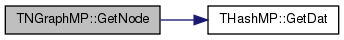Here is the caller graph for this function: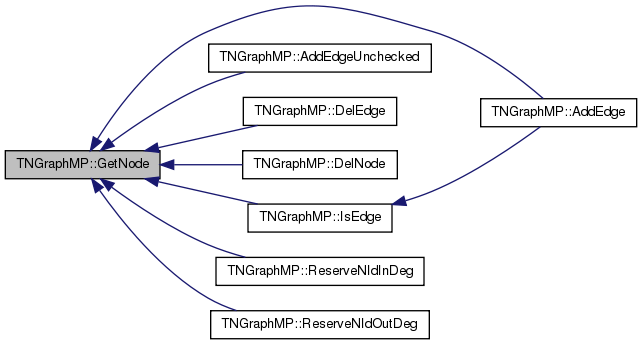const TNode& TNGraphMP::GetNode ( const int & NId ) const
inlineprivate

Definition at line 130 of file graphmp.h.

References THashMP< TKey, TDat, THashFunc >::GetDat().

130 { return NodeH.GetDat(NId); }
THashMP< TInt, TNode > NodeH
Definition: graphmp.h:127
const TDat & GetDat(const TKey &Key) const
Definition: hashmp.h:195

Here is the call graph for this function:int TNGraphMP::GetNodes ( ) const
inline

Returns the number of nodes in the graph.

Definition at line 154 of file graphmp.h.

References THashMP< TKey, TDat, THashFunc >::Len().

Referenced by Dump(), Empty(), and GetNIdV().

154 { return NodeH.Len(); }
THashMP< TInt, TNode > NodeH
Definition: graphmp.h:127
int Len() const
Definition: hashmp.h:165

Here is the call graph for this function: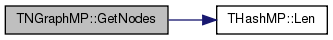Here is the caller graph for this function:TNodeI TNGraphMP::GetRndNI ( TRnd & Rnd = `TInt::Rnd` )
inline

Returns an interator referring to a random node in the graph.

Definition at line 250 of file graphmp.h.

References GetNI(), and GetRndNId().

250 { return GetNI(GetRndNId(Rnd)); }
TNodeI GetNI(const int &NId) const
Returns an iterator referring to the node of ID NId in the graph.
Definition: graphmp.h:201
int GetRndNId(TRnd &Rnd=TInt::Rnd)
Returns an ID of a random node in the graph.
Definition: graphmp.h:248

Here is the call graph for this function:int TNGraphMP::GetRndNId ( TRnd & Rnd = `TInt::Rnd` )
inline

Returns an ID of a random node in the graph.

Definition at line 248 of file graphmp.h.

Referenced by GetRndNI().

248 { return NodeH.GetKey(NodeH.GetRndKeyId(Rnd, 0.8)); }
int GetRndKeyId(TRnd &Rnd) const
Get an index of a random element. If the hash table has many deleted keys, this may take a long time...
Definition: hashmp.h:558
THashMP< TInt, TNode > NodeH
Definition: graphmp.h:127
const TKey & GetKey(const int &KeyId) const
Definition: hashmp.h:185

Here is the call graph for this function:Here is the caller graph for this function: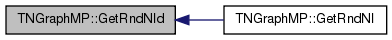PNGraphMP TNGraphMP::GetSmallGraph ( )
static

Returns a small graph on 5 nodes and 6 edges.

```/// Edges:  0 -> 1, 1 -> 2, 0 -> 2, 1 -> 3, 3 -> 4, 2 -> 3
/// ```

Definition at line 223 of file graphmp.cpp.

References New().

223  {
225  for (int i = 0; i < 5; i++) { G->AddNode(i); }
230  return G;
231 }
static PNGraphMP New()
Static constructor that returns a pointer to the graph. Call: PNGraphMP Graph = TNGraphMP::New().
Definition: graphmp.h:141
Definition: bd.h:196

Here is the call graph for this function: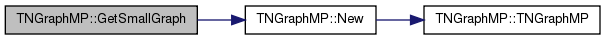bool TNGraphMP::HasFlag ( const TGraphFlag & Flag ) const

Allows for run-time checking the type of the graph (see the TGraphFlag for flags).

Definition at line 6 of file graphmp.cpp.

References HasGraphFlag.

6  {
7  return HasGraphFlag(TNGraphMP::TNet, Flag);
8 }
#define HasGraphFlag(TGraph, Flag)
For quick testing of the properties of the graph/network object (see TGraphFlag). ...
Definition: gbase.h:41
Definition: graphmp.h:27
 bool TNGraphMP::IsEdge ( const int & SrcNId, const int & DstNId, const bool & IsDir = `true` ) const

Tests whether an edge from node IDs SrcNId to DstNId exists in the graph.

Definition at line 130 of file graphmp.cpp.

References GetNode(), IsNode(), and TNGraphMP::TNode::IsOutNId().

130  {
131  if (! IsNode(SrcNId) || ! IsNode(DstNId)) { return false; }
132  if (IsDir) { return GetNode(SrcNId).IsOutNId(DstNId); }
133  else { return GetNode(SrcNId).IsOutNId(DstNId) || GetNode(DstNId).IsOutNId(SrcNId); }
134 }
bool IsNode(const int &NId) const
Tests whether ID NId is a node.
Definition: graphmp.h:195
bool IsOutNId(const int &NId) const
Definition: graphmp.h:50
TNode & GetNode(const int &NId)
Definition: graphmp.h:129

Here is the call graph for this function:Here is the caller graph for this function: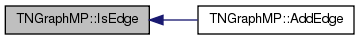bool TNGraphMP::IsNode ( const int & NId ) const
inline

Tests whether ID NId is a node.

Definition at line 195 of file graphmp.h.

References THashMP< TKey, TDat, THashFunc >::IsKey().

195 { return NodeH.IsKey(NId); }
bool IsKey(const TKey &Key) const
Definition: hashmp.h:191
THashMP< TInt, TNode > NodeH
Definition: graphmp.h:127

Here is the call graph for this function: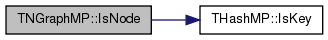Here is the caller graph for this function: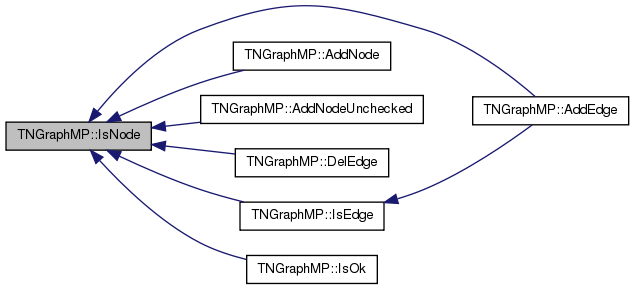bool TNGraphMP::IsOk ( const bool & ThrowExcept = `true` ) const

Checks the graph data structure for internal consistency.

For each node in the graph check that its neighbors are also nodes in the graph.

Definition at line 160 of file graphmp.cpp.

160  {
161  bool RetVal = true;
162  for (int N = NodeH.FFirstKeyId(); NodeH.FNextKeyId(N); ) {
163  const TNode& Node = NodeH[N];
164  if (! Node.OutNIdV.IsSorted()) {
165  const TStr Msg = TStr::Fmt("Out-neighbor list of node %d is not sorted.", Node.GetId());
166  if (ThrowExcept) { EAssertR(false, Msg); } else { ErrNotify(Msg.CStr()); } RetVal=false;
167  }
168  if (! Node.InNIdV.IsSorted()) {
169  const TStr Msg = TStr::Fmt("In-neighbor list of node %d is not sorted.", Node.GetId());
170  if (ThrowExcept) { EAssertR(false, Msg); } else { ErrNotify(Msg.CStr()); } RetVal=false;
171  }
172  // check out-edges
173  int prevNId = -1;
174  for (int e = 0; e < Node.GetOutDeg(); e++) {
175  if (! IsNode(Node.GetOutNId(e))) {
176  const TStr Msg = TStr::Fmt("Out-edge %d --> %d: node %d does not exist.",
177  Node.GetId(), Node.GetOutNId(e), Node.GetOutNId(e));
178  if (ThrowExcept) { EAssertR(false, Msg); } else { ErrNotify(Msg.CStr()); } RetVal=false;
179  }
180  if (e > 0 && prevNId == Node.GetOutNId(e)) {
181  const TStr Msg = TStr::Fmt("Node %d has duplidate out-edge %d --> %d.",
182  Node.GetId(), Node.GetId(), Node.GetOutNId(e));
183  if (ThrowExcept) { EAssertR(false, Msg); } else { ErrNotify(Msg.CStr()); } RetVal=false;
184  }
185  prevNId = Node.GetOutNId(e);
186  }
187  // check in-edges
188  prevNId = -1;
189  for (int e = 0; e < Node.GetInDeg(); e++) {
190  if (! IsNode(Node.GetInNId(e))) {
191  const TStr Msg = TStr::Fmt("In-edge %d <-- %d: node %d does not exist.",
192  Node.GetId(), Node.GetInNId(e), Node.GetInNId(e));
193  if (ThrowExcept) { EAssertR(false, Msg); } else { ErrNotify(Msg.CStr()); } RetVal=false;
194  }
195  if (e > 0 && prevNId == Node.GetInNId(e)) {
196  const TStr Msg = TStr::Fmt("Node %d has duplidate in-edge %d <-- %d.",
197  Node.GetId(), Node.GetId(), Node.GetInNId(e));
198  if (ThrowExcept) { EAssertR(false, Msg); } else { ErrNotify(Msg.CStr()); } RetVal=false;
199  }
200  prevNId = Node.GetInNId(e);
201  }
202  }
203  return RetVal;
204 }
void ErrNotify(const char *NotifyCStr)
Definition: bd.h:74
bool IsNode(const int &NId) const
Tests whether ID NId is a node.
Definition: graphmp.h:195
bool FNextKeyId(int &KeyId) const
Definition: hashmp.h:484
THashMP< TInt, TNode > NodeH
Definition: graphmp.h:127
Definition: dt.h:412
static TStr Fmt(const char *FmtStr,...)
Definition: dt.cpp:1599
int FFirstKeyId() const
Definition: hashmp.h:207
#define EAssertR(Cond, MsgStr)
Definition: bd.h:283
char * CStr()
Definition: dt.h:479

Here is the call graph for this function: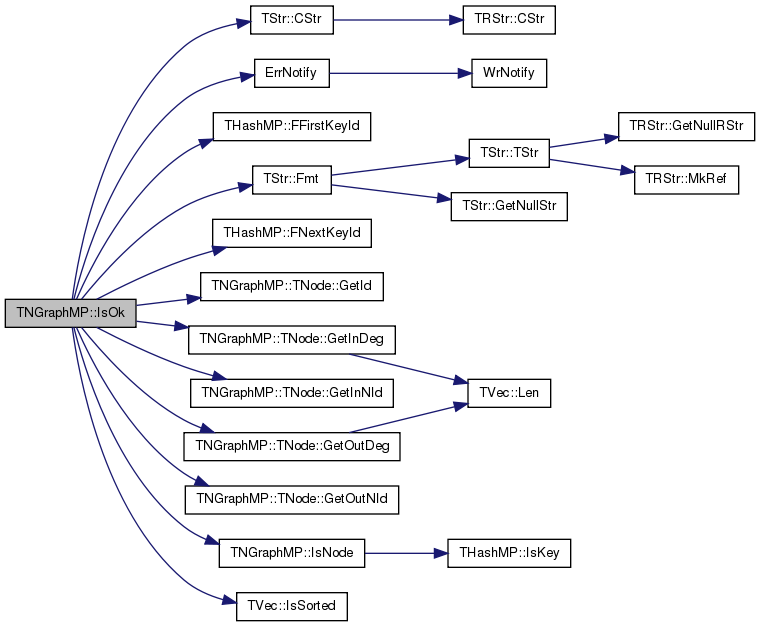static PNGraphMP TNGraphMP::Load ( TSIn & SIn )
inlinestatic

Static constructor that loads the graph from a stream SIn and returns a pointer to it.

Definition at line 147 of file graphmp.h.

References TNGraphMP().

147 { return PNGraphMP(new TNGraphMP(SIn)); }
TNGraphMP()
Definition: graphmp.h:132
TPt< TNGraphMP > PNGraphMP
Definition: graphmp.h:7

Here is the call graph for this function: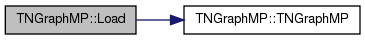static PNGraphMP TNGraphMP::New ( )
inlinestatic

Static constructor that returns a pointer to the graph. Call: PNGraphMP Graph = TNGraphMP::New().

Definition at line 141 of file graphmp.h.

References TNGraphMP().

Referenced by GetSmallGraph(), TSnap::ToGraphMP(), and TSnap::ToGraphMP3().

141 { return new TNGraphMP(); }
TNGraphMP()
Definition: graphmp.h:132

Here is the call graph for this function: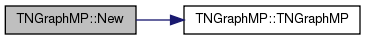Here is the caller graph for this function:static PNGraphMP TNGraphMP::New ( const int & Nodes, const int & Edges )
inlinestatic

Static constructor that returns a pointer to the graph and reserves enough memory for Nodes nodes and Edges edges.

Call: PNGraphMP Graph = TNGraphMP::New(Nodes, Edges).

Definition at line 145 of file graphmp.h.

References TNGraphMP().

145 { return new TNGraphMP(Nodes, Edges); }
TNGraphMP()
Definition: graphmp.h:132

Here is the call graph for this function:TNGraphMP& TNGraphMP::operator= ( const TNGraphMP & Graph )
inline

Definition at line 150 of file graphmp.h.

References MxNId, and NodeH.

150  {
151  if (this!=&Graph) { MxNId=Graph.MxNId; NodeH=Graph.NodeH; } return *this; }
THashMP< TInt, TNode > NodeH
Definition: graphmp.h:127
TInt MxNId
Definition: graphmp.h:126
 void TNGraphMP::Reserve ( const int & Nodes, const int & Edges )
inline

Reserves memory for a graph of Nodes nodes and Edges edges.

Definition at line 259 of file graphmp.h.

References THashMP< TKey, TDat, THashFunc >::Gen().

Referenced by TNGraphMP().

259 { if (Nodes>0) { NodeH.Gen(Nodes); } }
void Gen(const int &ExpectVals)
Definition: hashmp.h:160
THashMP< TInt, TNode > NodeH
Definition: graphmp.h:127

Here is the call graph for this function: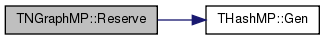Here is the caller graph for this function: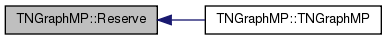int TNGraphMP::Reserved ( ) const
inline

Definition at line 206 of file graphmp.h.

206 {return NodeH.GetReservedKeyIds();}
int GetReservedKeyIds() const
Definition: hashmp.h:169
THashMP< TInt, TNode > NodeH
Definition: graphmp.h:127

Here is the call graph for this function: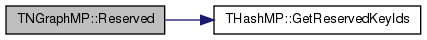Here is the caller graph for this function: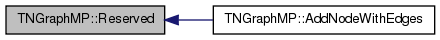void TNGraphMP::ReserveNIdInDeg ( const int & NId, const int & InDeg )
inline

Reserves memory for node ID NId having InDeg in-edges.

Definition at line 263 of file graphmp.h.

References GetNode(), TNGraphMP::TNode::InNIdV, and TVec< TVal, TSizeTy >::Reserve().

263 { GetNode(NId).InNIdV.Reserve(InDeg); }
TIntV InNIdV
Definition: graphmp.h:35
void Reserve(const TSizeTy &_MxVals)
Reserves enough memory for the vector to store _MxVals elements.
Definition: ds.h:543
TNode & GetNode(const int &NId)
Definition: graphmp.h:129

Here is the call graph for this function:void TNGraphMP::ReserveNIdOutDeg ( const int & NId, const int & OutDeg )
inline

Reserves memory for node ID NId having OutDeg out-edges.

Definition at line 265 of file graphmp.h.

References GetNode(), TNGraphMP::TNode::OutNIdV, and TVec< TVal, TSizeTy >::Reserve().

265 { GetNode(NId).OutNIdV.Reserve(OutDeg); }
TIntV OutNIdV
Definition: graphmp.h:35
void Reserve(const TSizeTy &_MxVals)
Reserves enough memory for the vector to store _MxVals elements.
Definition: ds.h:543
TNode & GetNode(const int &NId)
Definition: graphmp.h:129

Here is the call graph for this function:void TNGraphMP::ReserveNodeDegs ( const int & Idx, const int & InDeg, const int & OutDeg )
inline

Reserves memory for node Idx having InDeg in-edges and OutDeg out-edges.

Definition at line 261 of file graphmp.h.

261 { if (InDeg > 0) NodeH[Idx].InNIdV.Reserve(InDeg); if (OutDeg > 0) NodeH[Idx].OutNIdV.Reserve(OutDeg); }
THashMP< TInt, TNode > NodeH
Definition: graphmp.h:127
 void TNGraphMP::Save ( TSOut & SOut ) const
inline

Saves the graph to a (binary) stream SOut.

Definition at line 139 of file graphmp.h.

References THashMP< TKey, TDat, THashFunc >::Save(), and TInt::Save().

139 { MxNId.Save(SOut); NodeH.Save(SOut); }
void Save(TSOut &SOut) const
Definition: hashmp.h:129
void Save(TSOut &SOut) const
Definition: dt.h:1153
THashMP< TInt, TNode > NodeH
Definition: graphmp.h:127
TInt MxNId
Definition: graphmp.h:126

Here is the call graph for this function:void TNGraphMP::SetNodes ( const int & Length )
inline

Sets the number of nodes in the graph.

Definition at line 156 of file graphmp.h.

References THashMP< TKey, TDat, THashFunc >::SetLen().

156 { NodeH.SetLen(Length); }
void SetLen(const int &Length)
Definition: hashmp.h:166
THashMP< TInt, TNode > NodeH
Definition: graphmp.h:127

Here is the call graph for this function: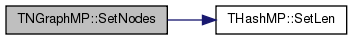void TNGraphMP::SortEdges ( const int & Idx, const int & InDeg, const int & OutDeg )
inline

Sorts in-edges and out-edges.

Definition at line 267 of file graphmp.h.

267 { if (InDeg > 0) NodeH[Idx].InNIdV.Sort(); if (OutDeg > 0) NodeH[Idx].OutNIdV.Sort(); }
THashMP< TInt, TNode > NodeH
Definition: graphmp.h:127
inline

Sorts the adjacency lists of each node.

Definition at line 269 of file graphmp.h.

References BegNI(), and EndNI().

269 { for (TNodeI NI = BegNI(); NI < EndNI(); NI++) { NI.SortNIdV();} }
TNodeI BegNI() const
Returns an iterator referring to the first node in the graph.
Definition: graphmp.h:197
TNodeI EndNI() const
Returns an iterator referring to the past-the-end node in the graph.
Definition: graphmp.h:199

Here is the call graph for this function:## Friends And Related Function Documentation

 friend class TNGraphMPMtx
friend

Definition at line 291 of file graphmp.h.

 friend class TPt< TNGraphMP >
friend

Definition at line 290 of file graphmp.h.

## Member Data Documentation

 TCRef TNGraphMP::CRef
private

Definition at line 125 of file graphmp.h.

 TInt TNGraphMP::MxNId
private

Definition at line 126 of file graphmp.h.